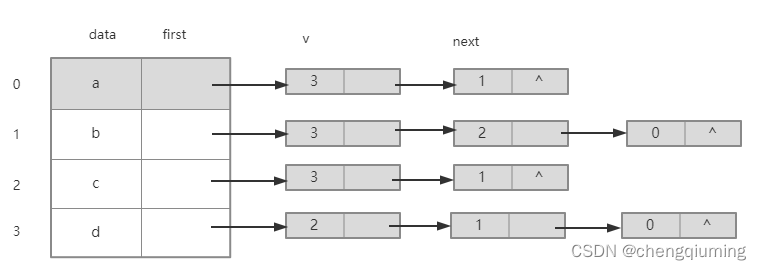# Java用鄰接表存儲圖的示例代碼

## 一、點睛

### 1.無向圖### 2.無向圖的鏈接表## 二、鄰接表的數據結構

### 1.節點### 2.鄰接點## 三、算法步驟

1 輸入節點數和邊數。

2 依次輸入節點信息，將其存儲到節點數組 Vex[] 的 data 域中，將 Vex[] first 域置空。

3 依次輸入每條邊依附的兩個節點，如果是網，則還需要輸入該邊的權值。

## 四、實現

```package graph;

import java.util.Scanner;

public class CreateALGraph {
static final int MaxVnum = 100;  // 頂點數最大值

public static void main(String[] args) {
ALGraph G = new ALGraph();
for (int i = 0; i < G.Vex.length; i++) {
G.Vex[i] = new VexNode();
}
CreateALGraph(G); // 創建有向圖鄰接表
printg(G); // 輸出鄰接表
}

static int locatevex(ALGraph G, char x) {
for (int i = 0; i < G.vexnum; i++) // 查找頂點信息的下標
if (x == G.Vex[i].data)
return i;
return -1; // 沒找到
}

// 插入一條邊
static void insertedge(ALGraph G, int i, int j) {
s.v = j;
s.next = G.Vex[i].first;
G.Vex[i].first = s;
}

// 輸出鄰接表
static void printg(ALGraph G) {
System.out.println("----------鄰接表如下：----------");

for (int i = 0; i < G.vexnum; i++) {
System.out.print(G.Vex[i].data + "：  ");
while (t != null) {
System.out.print("[" + t.v + "]\t");
t = t.next;
}
System.out.println();
}
}

// 創建有向圖鄰接表
static void CreateALGraph(ALGraph G) {
int i, j;
char u, v;

System.out.println("請輸入頂點數和邊數:");
Scanner scanner = new Scanner(System.in);
G.vexnum = scanner.nextInt();
G.edgenum = scanner.nextInt();
System.out.println("請輸入頂點信息:");

for (i = 0; i < G.vexnum; i++)//輸入頂點信息，存入頂點信息數組
G.Vex[i].data = scanner.next().charAt(0);
for (i = 0; i < G.vexnum; i++)
G.Vex[i].first = null;
System.out.println("請依次輸入每條邊的兩個頂點u,v");

while (G.edgenum-- > 0) {
u = scanner.next().charAt(0);
v = scanner.next().charAt(0);
i = locatevex(G, u); // 查找頂點 u 的存儲下標
j = locatevex(G, v); // 查找頂點 v 的存儲下標
if (i != -1 && j != -1)
insertedge(G, i, j);
else {
System.out.println("輸入頂點信息錯！請重新輸入！");
G.edgenum++; // 本次輸入不算
}
}
}
}

// 定義鄰接點類型
int v; // 鄰接點下標
}

// 定義頂點類型
class VexNode {
char data; // VexType為頂點的數據類型，根據需要定義
}

// 定義鄰接表類型
class ALGraph {
VexNode Vex[] = new VexNode[CreateALGraph.MaxVnum];
int vexnum; // 頂點數
int edgenum; // 邊數
}```

## 五、測試